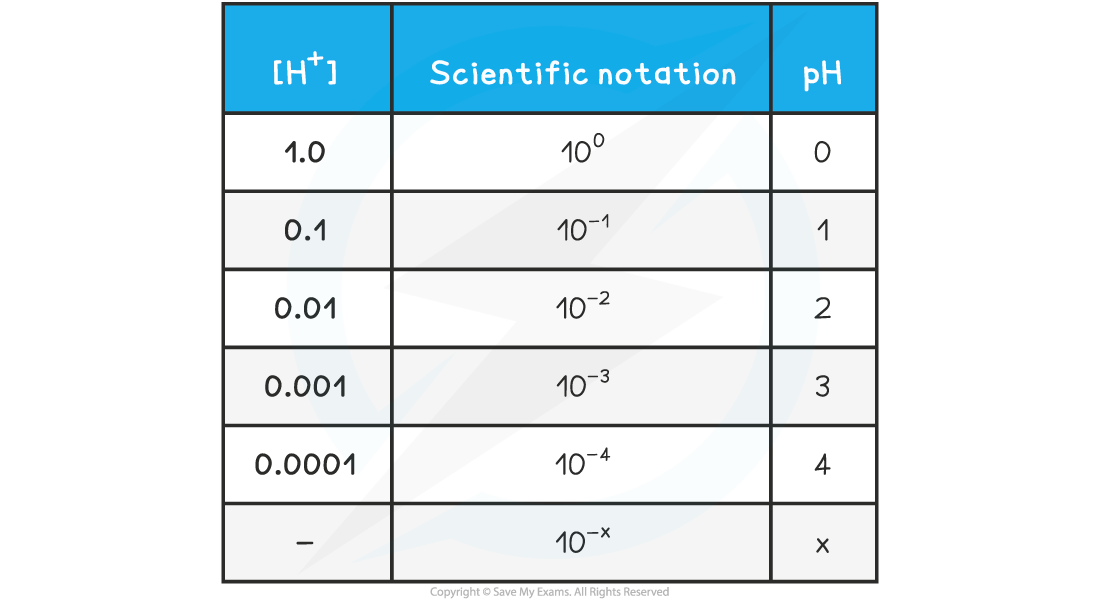# IB DP Chemistry: SL复习笔记8.2.2 pH & [H⁺]

### pH & [H⁺]

• The acidity of an aqueous solution depends on the number of H+ (H3O+) ions in solution
• The pH is defined as:• where [H+] is the concentration of H+ in mol dm–3
• The pH scale is a logarithmic scale with base 10
• This means that each value is 10 times the value below it. For example, pH 5 is 10 times more acidic than pH 6.
• pH values are usually given to 2 decimal places
• The relationship between concentration is easily seen on the following table:

pH & [H+] Table#### Worked Example

10.0 cmof an aqueous solution of nitric acid of pH = 1.0 is mixed with 990.0 cm3 of distilled water. What is the pH of the final solution?

A.  1

B.  2

C.  3

D.  10

The correct option is C.

• The total volume after dilution is 1000.0 cm3 so the concentration of H+ has been reduced by a factor of 100 or 10-2, which means an increase of 2 pH units
• The final solution is therefore pH 3

#### Exam Tip

Make sure you know how to use the antilog (base 10) feature on your calculator. On most calculators it is the 10x button, but on other models it could be LOG-1, ALOG or even a two-button sequence such as INV + LOG